# Dimensionality reduction and blind source separation¶

Requires HyperSpy v1.3 or above.

## Summary¶

This tutorial shows how to perform matrix factorization and blind source separation on spectra using HyperSpy. The same procedure can be used to analyse objects of higher dimensionality.

## Credits and changes¶

• 23/8/2016 Michael Walls. Extra explanations.
• 27/7/2016 Francisco de la Peña. Updated for HyperSpy v1.0.1.
• 6/3/2016 Francisco de la Peña. Adapted from previous tutorials for the SCANDEM workshop.

## 1. Singular value decomposition¶

This is a powerful method for reducing noise in hyperspectral datasets. Here we begin with a few lines of matrix algebra outlining the principles of the technique, but the demo can be followed easily without a full understanding of the mathematics. As with the "getting started" demo you can run this one interactively by downloading it and saving it as a .ipynb file.

Lets start by supposing a line-spectrum $D$ that can be described by a linear model.

$D=\left[d_{i,j}\right]_{m\times n}=\left[{\color{green}p_{i,j}}\right]_{m\times{\color{red}l}}\times}\left[{\color{red}{\color{blue}s_{i,j}}}\right]_{{\color{red}l}\times n$ where $m$ is the number of pixels in the line scan, $n$ the number of channels in the spectrum and $l$ the number of components e.g. spectra of individual compounds.

Normally, what we actually measure is a noisy version of $D$, $D'$,

$D'=\left[d'_{i,j}\right]_{m\times n}=\left[{\color{green}p_{i,j}}\right]_{m\times{\color{red}l}}\times}\left[{\color{red}{\color{blue}s_{i,j}}}\right]_{{\color{red}l}\times n}+\mathrm{Noise$

$D'$ could be factorized as follows:

$D'=\left[{\tilde{\color{green}p}}_{{i,j}}\right]_{m\times{\color{red}k}}\times}\left[\tilde{s}_{i,j}\right]_{{\color{red}k}\times n}$ where $k\leq\min\left(m,n\right)$.

Extra constraints are needed to fully determine the matrix factorization. When we add the orthogonality constraint we refer to this decomposition as singular value decomposition (SVD).

In our assumption of a linear model:

$D'=\left[{\tilde{\color{green}p}}_{{i,j}}\right]_{m\times{\color{red}l}}\times}\left[\tilde{s}_{i,j}\right]_{{\color{red}l}\times n}+ \left[{\tilde{\color{green}p}}_{{i,j}}\right]_{m\times{\color{red}{k-l}}}\times}\left[\tilde{s}_{i,j}\right]_{{\color{red}{k-l}}\times n$

With

$D\approx\left[{\tilde{\color{green}p}}_{{i,j}}\right]_{m\times{\color{red}l}}\times}\left[\tilde{s}_{i,j}\right]_{{\color{red}l}\times n$

$\mathrm{Noise}\approx\left[{\tilde{\color{green}p}}_{{i,j}}\right]_{m\times{\color{red}{k-l}}}\times}\left[\tilde{s}_{i,j}\right]_{{\color{red}{k-l}}\times n$

In :
from urllib.request import urlretrieve, urlopen
from zipfile import ZipFile
files = urlretrieve("https://www.dropbox.com/s/dt6bc3dtg373ahw/machine_learning.zip?raw=1", "./machine_learning.zip")

with ZipFile("machine_learning.zip") as z:
z.extractall()


### Set up matplotlib and import Hyperspy¶

NOTE: In the online version of this document we use the inline backend that displays interactive figures inside the Jupyter Notebook. However, for interactive data analysis purposes most would prefer to use the qt4, wx or nbagg backends.

In :
%matplotlib nbagg
# or qt4 etc
import hyperspy.api as hs

WARNING:hyperspy_gui_traitsui:The nbAgg matplotlib backend is not supported by the installed traitsui version and the ETS toolkit has been set to null. To set the ETS toolkit independently from the matplotlib backend, set it before importing matplotlib.
WARNING:hyperspy_gui_traitsui:The traitsui GUI elements are not available.


In :
s = hs.load("machine_learning/CL1.hdf5")


This is a synthetic electron energy-loss spectroscopy dataset. The procedure, although not fully general, can easily be used as is or with minor adaptation to analyse other kinds of data including images and higher dimensional signals.

In :
s.plot()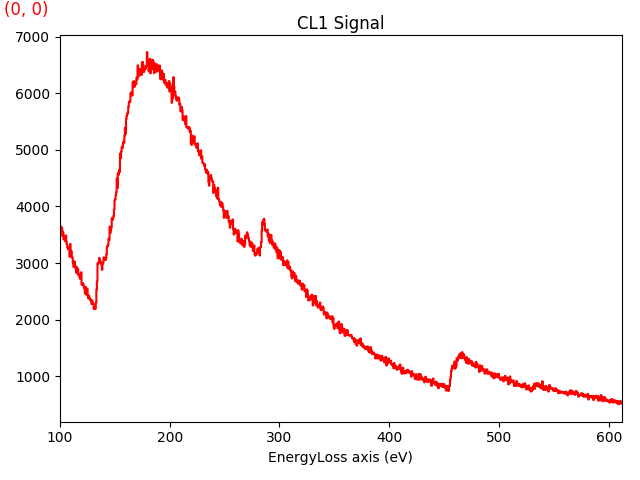To perform SVD in HyperSpy we use the decomposition method that, by default, performs SVD.

In :
s.decomposition()


The result of the decomposition is stored in the learning_results attribute.

In :
s.learning_results.summary()

Decomposition parameters:
-------------------------

Decomposition algorithm : 	svd
Poissonian noise normalization : False
Output dimension : None
Centre : None


SVD decomposes the data in so-called "components" and sorts them in order of decreasing relevance. It is often useful to estimate the dimensionality of the data by plotting the explained variance against the component index on a logarithmic y-scale. This plot is sometimes called a scree-plot and it should drop quickly, eventually becoming a slowly descending line. The point at which it becomes linear is often a good estimation of the dimensionality of the data (or equivalently, the number of components that should be retained).

To plot the scree plot, run the plot_explained_variance_ratio method e.g.:

In :
s.plot_explained_variance_ratio()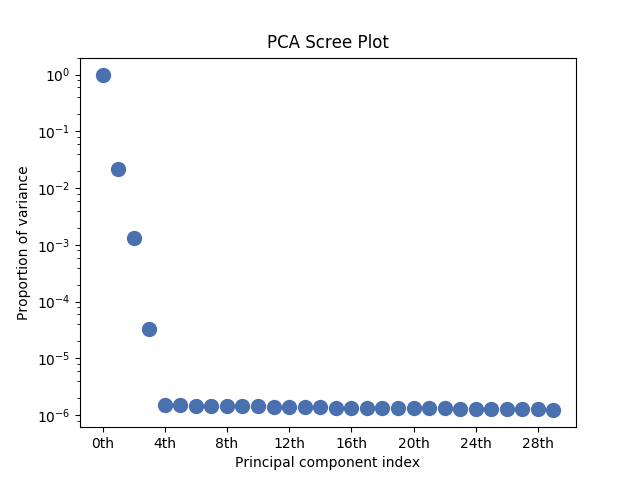Out:
<matplotlib.axes._subplots.AxesSubplot at 0x7f16554ca400>

From the scree plot we estimate that there are 4 principal components.

We can store the scree plot as a Spectrum instance using the following method:

In :
scree_plot = s.get_explained_variance_ratio()


### Shot noise¶

PCA assumes gaussian noise, however, the noise in EELS spectra is approximately poissonian (shot noise). It is possible to approximately "normalise" the noise by using a liner transformation, which should result in a better decomposition of data with shot noise. This is done in HyperSpy as follows:

In :
s.decomposition(normalize_poissonian_noise=True)


Let's plot the scree plot of this and the previous decomposition in the same figure

In :
ax = hs.plot.plot_spectra([scree_plot.isig[:20],
s.get_explained_variance_ratio().isig[:20]],
legend=("Std", "Normalized"))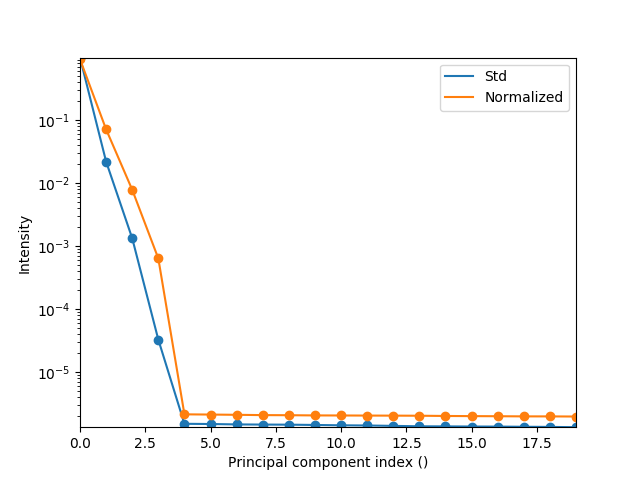Let's improve the plot using some matplotlib commands:

In :
ax.set_yscale('log')
ax.lines.set_marker("o")
ax.lines.set_marker("o")
ax.figure #for details of how these commands work see the matplotlib webpageAs we can see, the explained variance of the first four components of the normalized decomposition are "further away" from the noise than those of the original data, indicating a better decomposition.

### Visualise the decomposition results¶

The following commands can be used to plot the first $n$ principal components. (Note: the _= part at the beginning is for webpage rendering purposes and is not strictly needed in the command)

In :
_ = s.plot_decomposition_loadings(4)
_ = s.plot_decomposition_factors(4, comp_label="")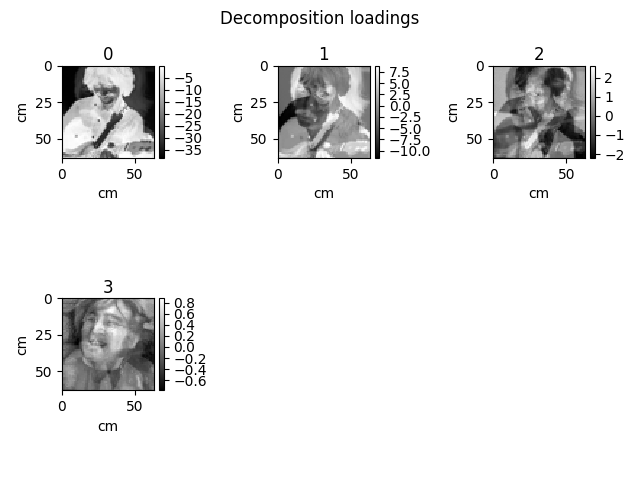/home/fjd29/Python/hyperspy3/hyperspy/signal.py:1511: VisibleDeprecationWarning: The 'comp_label' argument will be deprecated in 2.0, please use 'title' instead
VisibleDeprecationWarning)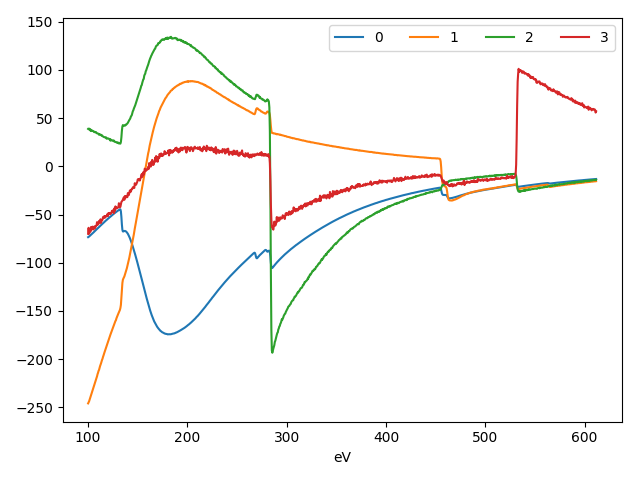Alternatively (and usually more conveniently) we can use the following plotting method. Use the slider or the left/right arrow keys to change the index of the components in interactive plotting mode.

In :
s.plot_decomposition_results()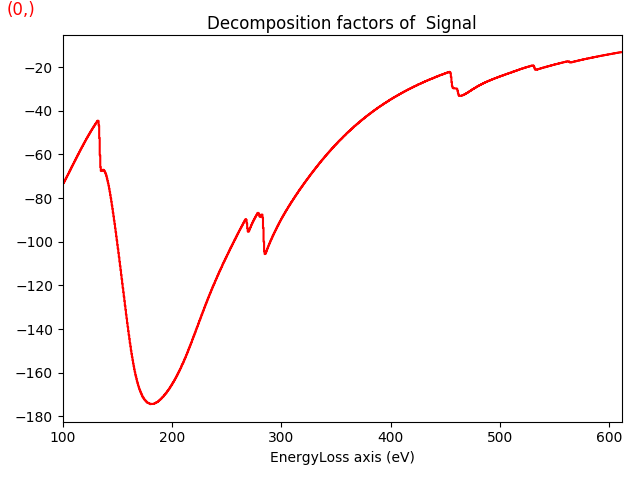### Noise reduction¶

A common application of PCA is noise reduction, which is achieved by dimensionality reduction. We can create a "denoised" version of the dataset by inverting the decomposition using only the number of principal components that we want to retain (in this case the first 4 components). This is done with the get_decomposition_model command.

In :
sc = s.get_decomposition_model(4)


Let's plot the spectra at coordinates (30,30) from the original and PCA-denoised datasets

In :
(s + sc * 1j).plot()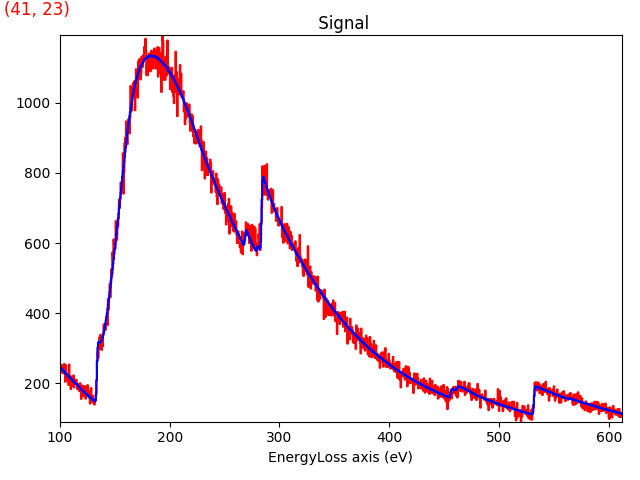Calculating and plotting the residuals at a given position can be done in one single line

In :
(s - sc).inav[30,30].plot()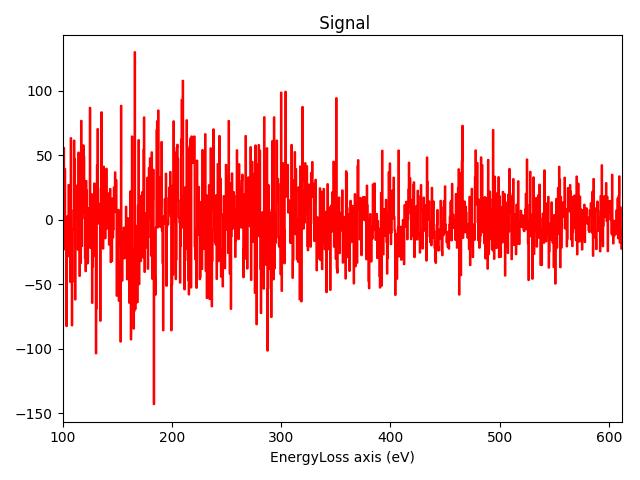## 2. Blind source separation¶

### Independent component analysis¶

As we have seen in the previous section, the principal components are a linear mixture of EELS elemental maps and spectra, but the mixing matrix is unknown. We can use blind source separation (BSS) to estimate the mixing matrix. In this case we will use independent component analysis. BSS is performed in HyperSpy using the blind_source_separation method that by default uses "FastICA" which is a well-tested ICA routine.

In :
s.blind_source_separation(4, diff_order=1)


The results are also stored in the learning_results attribute:

In :
s.learning_results.summary()

Decomposition parameters:
-------------------------

Decomposition algorithm : 	svd
Poissonian noise normalization : True
Output dimension : None
Centre : None

Demixing parameters:
------------------------
BSS algorithm : sklearn_fasticaNumber of components : 4


And can be visualised with the following commands:

In :
_ = s.plot_bss_loadings()
_ = s.plot_bss_factors()